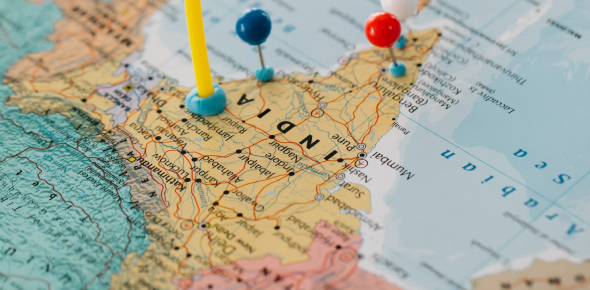# State Capitals Of India

30 Questions | Attempts: 6059
ShareSettings• 1.
Kolkata is the capital of which state?
• 2.
What is the capital of Uttarakhand?
• 3.
What is the capital of Uttar Pradesh?
• 4.
What is the capital of Tripura
• 5.
What is the capital of Tamil Nadu?
• 6.
Gantok is the capital of which state?
• 7.
What is the capital of Rajasthan?
• 8.
What is the capital of Nagaland?
• 9.
What is the capital of Odisha?
• 10.
What is the capital of Punjab?
• 11.
What is the capital of Jammu and Kashmir?
• 12.
What is the capital of Telangana?
• 13.
What is the capital of Jharkhand?
• 14.
What is the capital of Karnataka?
• 15.
What is the capital of Kerala?
• 16.
• 17.
What is the capital of Maharashtra?
• 18.
What is the capital of Manipur?
• 19.
What is the capital of Meghalaya?
• 20.
What is the capital of Mizoram?
• 21.
What is the capital of Seemandhra?
• 22.
What is the capital of Assam?
• 23.
What is the capital of Bihar?
• 24.
What is the capital of Chhattisgarh?
• 25.
What is the capital of Goa?

## Related TopicsBack to top
×

Wait!
Here's an interesting quiz for you.